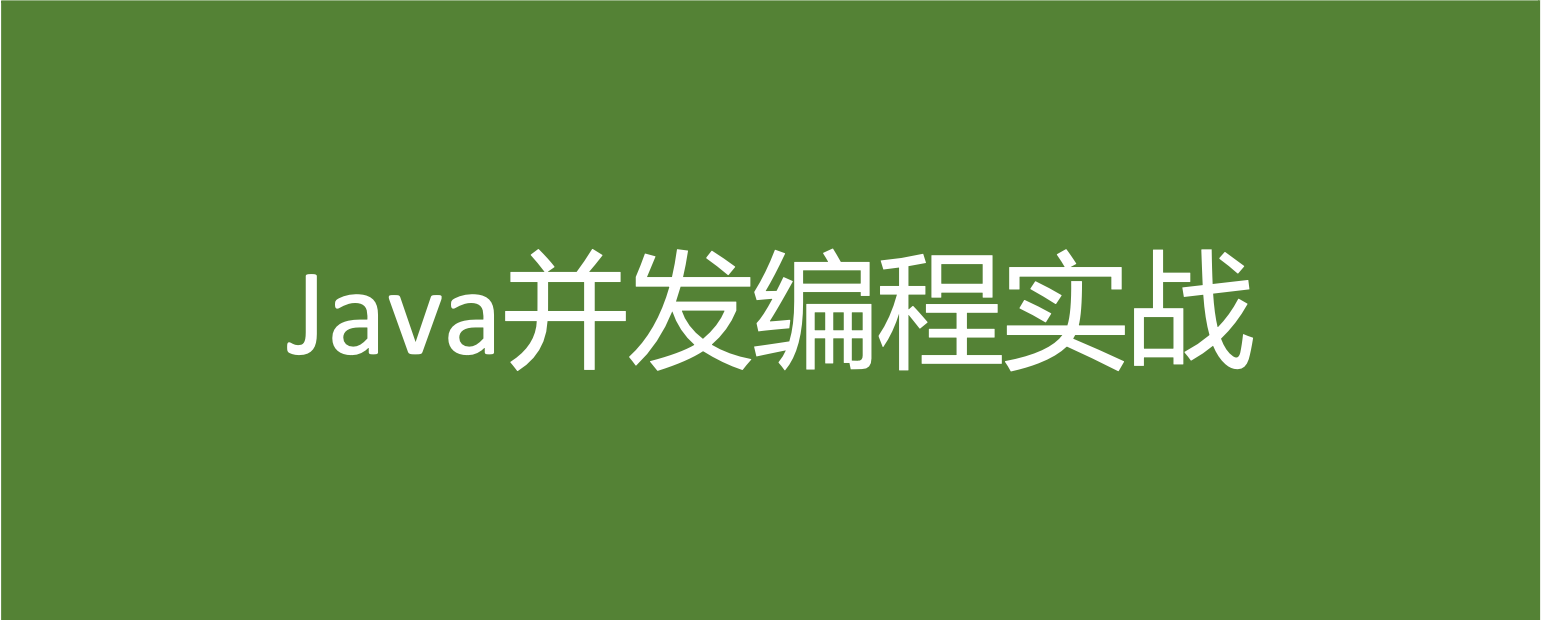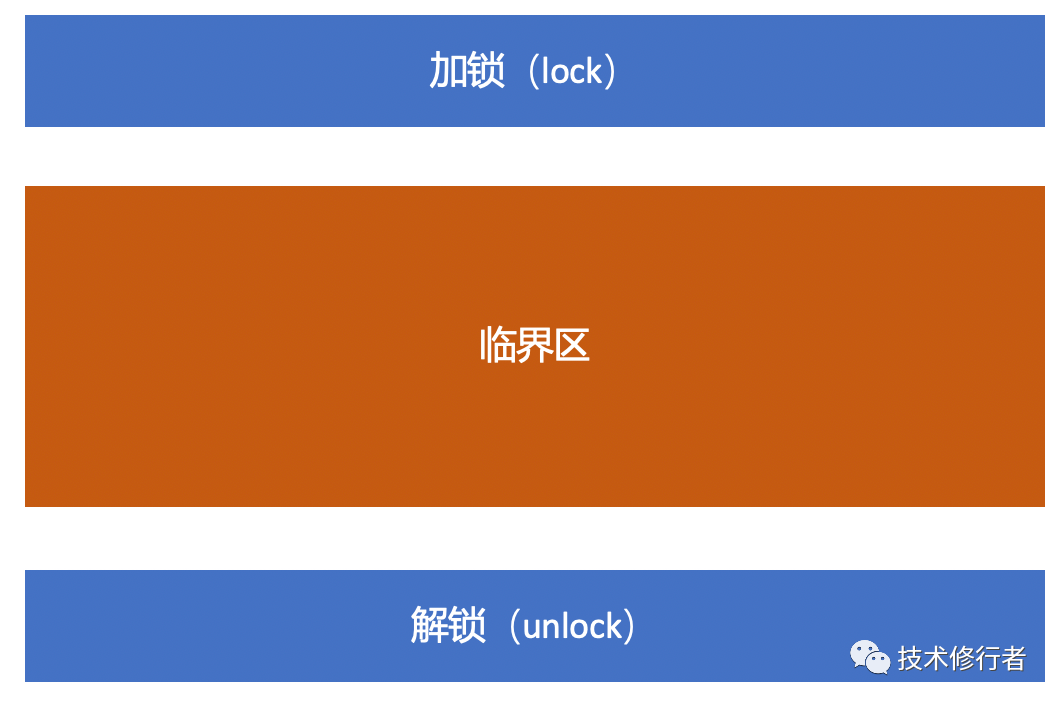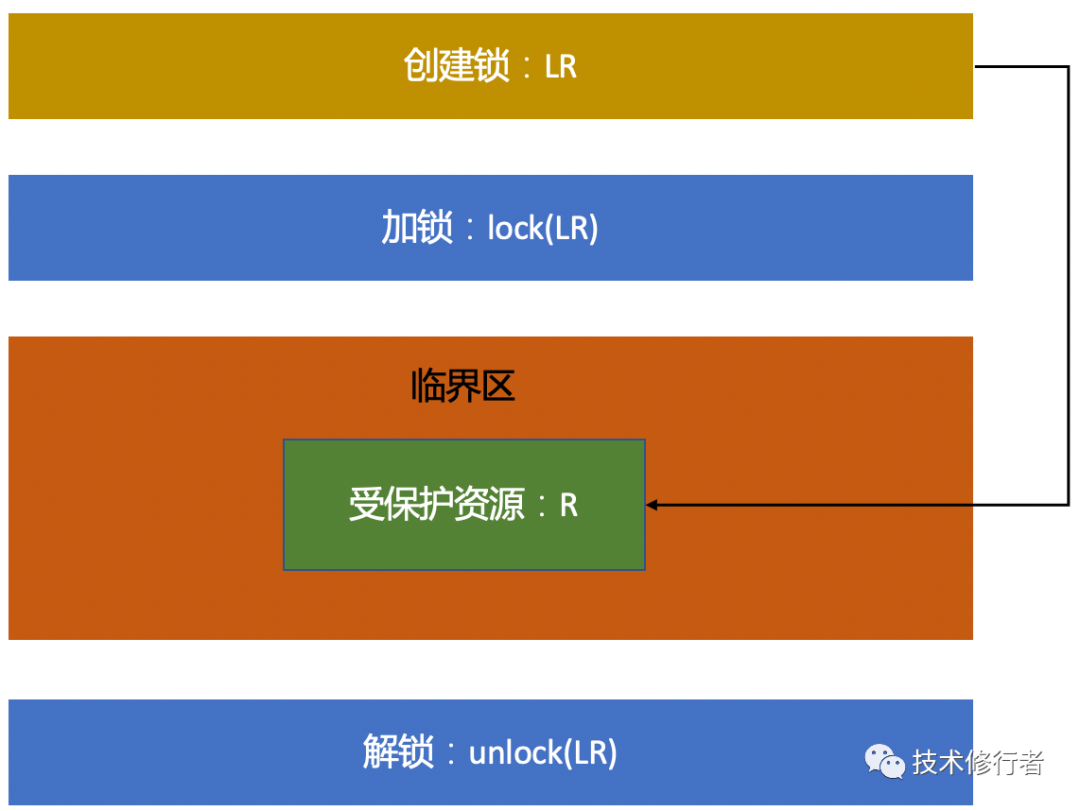# Java 并发编程实战（3）- 互斥锁# 互斥锁模型

## 互斥锁简易模型## 互斥锁改进模型

• 我们锁的是什么？

• 我们保护的又是什么？# Java 世界中的互斥锁

synchronized 关键字可以应用在方法上，也可以直接应用在代码块中。

public class SynchronizedDemo {	// 修饰实例方法	synchronized void updateData() {		// 业务代码	}		// 修饰静态方法	synchronized static void retrieveData() {		// 业务代码	}		// 修饰代码块	Object obj = new Object();		void createData() {		synchronized(obj) {			// 业务代码		}	}}

## synchronized 中的锁和锁对象

• 当修饰静态方法时，锁定的是当前类的 Class 对象。

• 当修饰非静态方法时，锁定的是当前实例对象 this。

// 修饰实例方法	synchronized void updateData() {		// 业务代码	}		// 修饰实例方法	synchronized(this) void updateData2() {		// 业务代码	}

	// 修饰静态方法	synchronized static void retrieveData() {		// 业务代码	}		// 修饰静态方法	synchronized(SynchronizedDemo.class) static void retrieveData2() {		// 业务代码	}

## synchronized 示例

public class ConcurrencySafeAddDemo {	private long count = 0;	private synchronized void safeAdd() {		int index = 0;		while (index < 10000) {			count = count + 1;			index++;		}	}	private void reset() {		this.count = 0;	}	private void addTest() throws InterruptedException {		List<Thread> threads = new ArrayList<Thread>();		for (int i = 0; i < 6; i++) {			threads.add(new Thread(() -> {				this.safeAdd();			}));		}				for (Thread thread : threads) {			thread.start();		}				for (Thread thread : threads) {			thread.join();		}				threads.clear();		System.out.println(String.format("Count is %s", count));	}	public static void main(String[] args) throws InterruptedException {		ConcurrencySafeAddDemo demoObj = new ConcurrencySafeAddDemo();		for (int i = 0; i < 10; i++) {			demoObj.addTest();			demoObj.reset();		}	}}

Count is 60000Count is 60000Count is 60000Count is 60000Count is 60000Count is 60000Count is 60000Count is 60000Count is 60000Count is 60000

# 锁与受保护的资源的关系

• 一个锁可以应用到多个受保护资源

• 一个受保护资源上只能有一个锁

	private void safeAdd() {		int index = 0;		synchronized(new Object()) {			while (index < 10000) {				count = count + 1;				index++;			}		}	}

Count is 17355Count is 18215Count is 19244Count is 20863Count is 60000Count is 60000Count is 60000Count is 20430Count is 60000Count is 60000

# 一个锁保护多个资源

## 一个锁保护多个没有关联关系的资源

public class BankAccountLockDemo {	private double balance;	private String password;		private Object commonLockObj = new Object();		// 取钱	private void withdrawMoney(double amount) {		synchronized(commonLockObj) {			// 业务代码			balance = balance - amount;		}	}		// 修改密码	private void changePassword(String newPassword) {		synchronized(commonLockObj) {			// 业务代码			password = newPassword;		}	}}

## 一个锁保护多个有关联关系的资源

public class BankAccountTransferLockDemo {	private double balance;		private Object lockObj = new Object();		private void transfer(BankAccountTransferLockDemo sourceAccount, BankAccountTransferLockDemo targetAccount, double amount) {		synchronized(lockObj) {			sourceAccount.balance = sourceAccount.balance - amount;			targetAccount.balance = targetAccount.balance + amount;		}	}}

	private void transfer(BankAccountTransferLockDemo sourceAccount, BankAccountTransferLockDemo targetAccount, double amount) {		synchronized(BankAccountTransferLockDemo.class) {			sourceAccount.balance = sourceAccount.balance - amount;			targetAccount.balance = targetAccount.balance + amount;		}	}

“原子性”的本质什么？## 评论